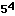Eric

I want to know why the grade six mathbooks tell you that little number at the top right of another number (exponent)is called the power,but in the grade seven and up books they tell you it's called the exponent?

Eric:

The language here can be confusing. The correct usage is to say thatis "five to the fourth power" or "the fourth power of five". So the fourth power of five is 625. The number 4 here is the called the exponent.

Harley

Go to Math Central